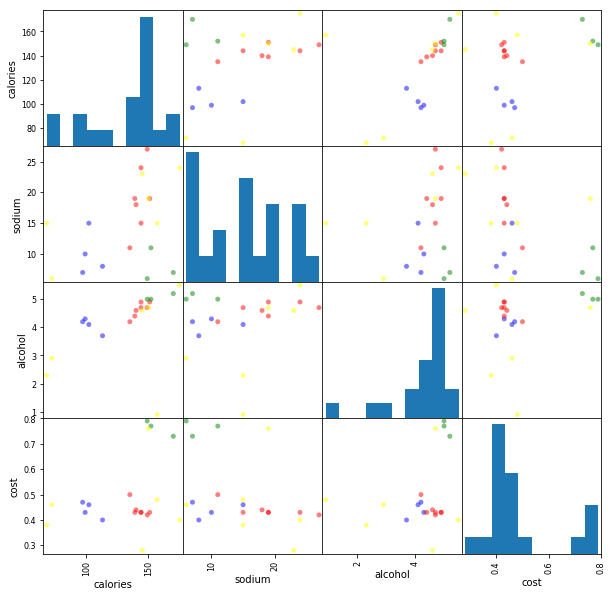# Clustering¶

## Agenda:¶

1. K-means clustering
2. Clustering evaluation
3. DBSCAN clustering
In :
# beer dataset
import pandas as pd
beer

Out:
name calories sodium alcohol cost
0 Budweiser 144 15 4.7 0.43
1 Schlitz 151 19 4.9 0.43
2 Lowenbrau 157 15 0.9 0.48
3 Kronenbourg 170 7 5.2 0.73
4 Heineken 152 11 5.0 0.77
5 Old_Milwaukee 145 23 4.6 0.28
6 Augsberger 175 24 5.5 0.40
7 Srohs_Bohemian_Style 149 27 4.7 0.42
8 Miller_Lite 99 10 4.3 0.43
9 Budweiser_Light 113 8 3.7 0.40
10 Coors 140 18 4.6 0.44
11 Coors_Light 102 15 4.1 0.46
12 Michelob_Light 135 11 4.2 0.50
13 Becks 150 19 4.7 0.76
14 Kirin 149 6 5.0 0.79
15 Pabst_Extra_Light 68 15 2.3 0.38
16 Hamms 139 19 4.4 0.43
17 Heilemans_Old_Style 144 24 4.9 0.43
18 Olympia_Goled_Light 72 6 2.9 0.46
19 Schlitz_Light 97 7 4.2 0.47

How would you cluster these beers?

In :
# define X
X = beer.drop('name', axis=1)


What happened to y?

## Part 1: K-means clustering¶

In :
# K-means with 3 clusters
from sklearn.cluster import KMeans
km = KMeans(n_clusters=3, random_state=1)
km.fit(X)

Out:
KMeans(algorithm='auto', copy_x=True, init='k-means++', max_iter=300,
n_clusters=3, n_init=10, n_jobs=1, precompute_distances='auto',
random_state=1, tol=0.0001, verbose=0)
In :
# review the cluster labels
km.labels_

Out:
array([0, 0, 0, 0, 0, 0, 0, 0, 1, 1, 0, 1, 0, 0, 0, 2, 0, 0, 2, 1],
dtype=int32)
In :
# save the cluster labels and sort by cluster
beer['cluster'] = km.labels_
beer.sort_values('cluster')

Out:
name calories sodium alcohol cost cluster
0 Budweiser 144 15 4.7 0.43 0
1 Schlitz 151 19 4.9 0.43 0
2 Lowenbrau 157 15 0.9 0.48 0
3 Kronenbourg 170 7 5.2 0.73 0
4 Heineken 152 11 5.0 0.77 0
5 Old_Milwaukee 145 23 4.6 0.28 0
6 Augsberger 175 24 5.5 0.40 0
7 Srohs_Bohemian_Style 149 27 4.7 0.42 0
17 Heilemans_Old_Style 144 24 4.9 0.43 0
16 Hamms 139 19 4.4 0.43 0
10 Coors 140 18 4.6 0.44 0
14 Kirin 149 6 5.0 0.79 0
12 Michelob_Light 135 11 4.2 0.50 0
13 Becks 150 19 4.7 0.76 0
9 Budweiser_Light 113 8 3.7 0.40 1
8 Miller_Lite 99 10 4.3 0.43 1
11 Coors_Light 102 15 4.1 0.46 1
19 Schlitz_Light 97 7 4.2 0.47 1
15 Pabst_Extra_Light 68 15 2.3 0.38 2
18 Olympia_Goled_Light 72 6 2.9 0.46 2

What do the clusters seem to be based on? Why?

In :
# review the cluster centers
km.cluster_centers_

Out:
array([[150.        ,  17.        ,   4.52142857,   0.52071429],
[102.75      ,  10.        ,   4.075     ,   0.44      ],
[ 70.        ,  10.5       ,   2.6       ,   0.42      ]])
In :
# calculate the mean of each feature for each cluster
beer.groupby('cluster').mean()

Out:
calories sodium alcohol cost
cluster
0 150.00 17.0 4.521429 0.520714
1 102.75 10.0 4.075000 0.440000
2 70.00 10.5 2.600000 0.420000
In :
# save the DataFrame of cluster centers
centers = beer.groupby('cluster').mean()

In :
# allow plots to appear in the notebook
%matplotlib inline
import matplotlib.pyplot as plt
plt.rcParams['font.size'] = 14

In :
# create a "colors" array for plotting
import numpy as np
colors = np.array(['red', 'green', 'blue', 'yellow'])

In :
# scatter plot of calories versus alcohol, colored by cluster (0=red, 1=green, 2=blue)
plt.scatter(beer.calories, beer.alcohol, c=colors[beer.cluster], s=50)

# cluster centers, marked by "+"
plt.scatter(centers.calories, centers.alcohol, linewidths=3, marker='+', s=300, c='black')

plt.xlabel('calories')
plt.ylabel('alcohol')

Out:
Text(0,0.5,'alcohol')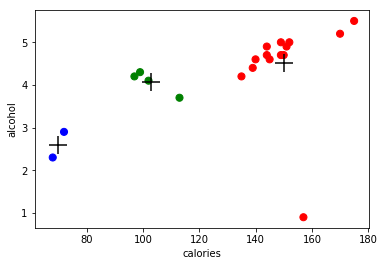In :
# scatter plot matrix (0=red, 1=green, 2=blue)
pd.scatter_matrix(X, c=colors[beer.cluster], figsize=(10,10), s=100)

/Users/georgioskarakostas/anaconda3/lib/python3.6/site-packages/ipykernel_launcher.py:2: FutureWarning: pandas.scatter_matrix is deprecated, use pandas.plotting.scatter_matrix instead


Out:
array([[<matplotlib.axes._subplots.AxesSubplot object at 0x1a17c78518>,
<matplotlib.axes._subplots.AxesSubplot object at 0x1a17cd1390>,
<matplotlib.axes._subplots.AxesSubplot object at 0x1a17d5d9b0>,
<matplotlib.axes._subplots.AxesSubplot object at 0x1a17d85f98>],
[<matplotlib.axes._subplots.AxesSubplot object at 0x1a17db5668>,
<matplotlib.axes._subplots.AxesSubplot object at 0x1a17db56a0>,
<matplotlib.axes._subplots.AxesSubplot object at 0x1a17e103c8>,
<matplotlib.axes._subplots.AxesSubplot object at 0x1a17e37a58>],
[<matplotlib.axes._subplots.AxesSubplot object at 0x1a17e6a128>,
<matplotlib.axes._subplots.AxesSubplot object at 0x1a17e917b8>,
<matplotlib.axes._subplots.AxesSubplot object at 0x1a17eb8e48>,
<matplotlib.axes._subplots.AxesSubplot object at 0x1a17eeb518>],
[<matplotlib.axes._subplots.AxesSubplot object at 0x1a17f14ba8>,
<matplotlib.axes._subplots.AxesSubplot object at 0x1a17f46278>,
<matplotlib.axes._subplots.AxesSubplot object at 0x1a17f6e908>,
<matplotlib.axes._subplots.AxesSubplot object at 0x1a17f96f98>]],
dtype=object)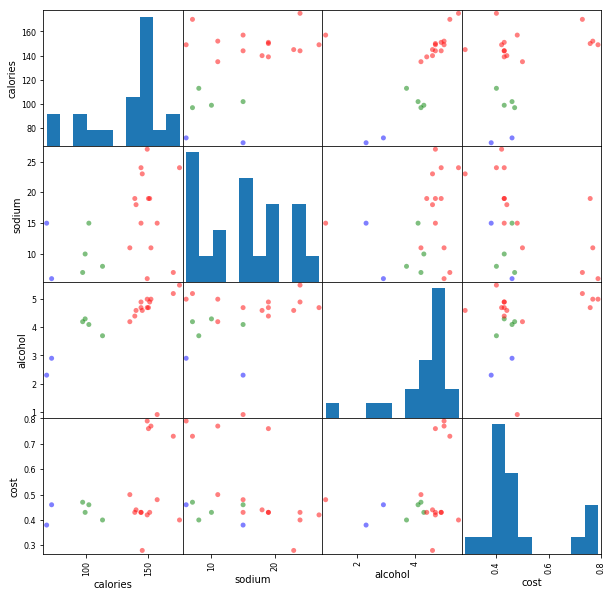### Repeat with scaled data¶

In :
# center and scale the data
from sklearn.preprocessing import StandardScaler
scaler = StandardScaler()
X_scaled = scaler.fit_transform(X)

In :
# K-means with 3 clusters on scaled data
km = KMeans(n_clusters=3, random_state=1)
km.fit(X_scaled)

Out:
KMeans(algorithm='auto', copy_x=True, init='k-means++', max_iter=300,
n_clusters=3, n_init=10, n_jobs=1, precompute_distances='auto',
random_state=1, tol=0.0001, verbose=0)
In :
# save the cluster labels and sort by cluster
beer['cluster'] = km.labels_
beer.sort_values('cluster')

Out:
name calories sodium alcohol cost cluster
0 Budweiser 144 15 4.7 0.43 0
1 Schlitz 151 19 4.9 0.43 0
17 Heilemans_Old_Style 144 24 4.9 0.43 0
16 Hamms 139 19 4.4 0.43 0
5 Old_Milwaukee 145 23 4.6 0.28 0
6 Augsberger 175 24 5.5 0.40 0
7 Srohs_Bohemian_Style 149 27 4.7 0.42 0
10 Coors 140 18 4.6 0.44 0
15 Pabst_Extra_Light 68 15 2.3 0.38 1
12 Michelob_Light 135 11 4.2 0.50 1
11 Coors_Light 102 15 4.1 0.46 1
9 Budweiser_Light 113 8 3.7 0.40 1
8 Miller_Lite 99 10 4.3 0.43 1
2 Lowenbrau 157 15 0.9 0.48 1
18 Olympia_Goled_Light 72 6 2.9 0.46 1
19 Schlitz_Light 97 7 4.2 0.47 1
13 Becks 150 19 4.7 0.76 2
14 Kirin 149 6 5.0 0.79 2
4 Heineken 152 11 5.0 0.77 2
3 Kronenbourg 170 7 5.2 0.73 2

What are the "characteristics" of each cluster?

In :
# review the cluster centers
beer.groupby('cluster').mean()

Out:
calories sodium alcohol cost
cluster
0 148.375 21.125 4.7875 0.4075
1 105.375 10.875 3.3250 0.4475
2 155.250 10.750 4.9750 0.7625
In :
# scatter plot matrix of new cluster assignments (0=red, 1=green, 2=blue)
pd.scatter_matrix(X, c=colors[beer.cluster], figsize=(10,10), s=100)

/Users/georgioskarakostas/anaconda3/lib/python3.6/site-packages/ipykernel_launcher.py:2: FutureWarning: pandas.scatter_matrix is deprecated, use pandas.plotting.scatter_matrix instead


Out:
array([[<matplotlib.axes._subplots.AxesSubplot object at 0x1a180123c8>,
<matplotlib.axes._subplots.AxesSubplot object at 0x1a183af630>,
<matplotlib.axes._subplots.AxesSubplot object at 0x1a18535cc0>,
<matplotlib.axes._subplots.AxesSubplot object at 0x1a18565390>],
[<matplotlib.axes._subplots.AxesSubplot object at 0x1a1858ea20>,
<matplotlib.axes._subplots.AxesSubplot object at 0x1a1858ea58>,
<matplotlib.axes._subplots.AxesSubplot object at 0x1a185e7780>,
<matplotlib.axes._subplots.AxesSubplot object at 0x1a18610e10>],
[<matplotlib.axes._subplots.AxesSubplot object at 0x1a186414e0>,
<matplotlib.axes._subplots.AxesSubplot object at 0x1a18669b70>,
<matplotlib.axes._subplots.AxesSubplot object at 0x1a1869b240>,
<matplotlib.axes._subplots.AxesSubplot object at 0x1a186c48d0>],
[<matplotlib.axes._subplots.AxesSubplot object at 0x1a186eff60>,
<matplotlib.axes._subplots.AxesSubplot object at 0x1a1871e630>,
<matplotlib.axes._subplots.AxesSubplot object at 0x1a18746cc0>,
<matplotlib.axes._subplots.AxesSubplot object at 0x1a18776400>]],
dtype=object)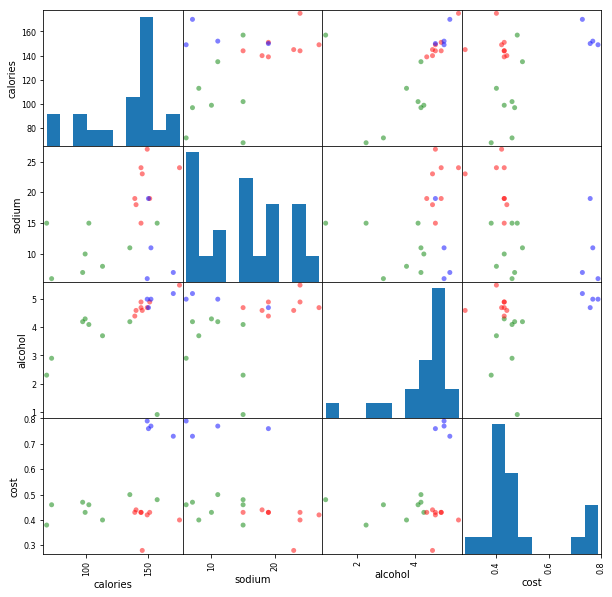Do you notice any cluster assignments that seem a bit odd? How might we explain those?

## Part 2: Clustering evaluation¶

The Silhouette Coefficient is a common metric for evaluating clustering "performance" in situations when the "true" cluster assignments are not known.

A Silhouette Coefficient is calculated for each observation:

$$SC = \frac{b-a} {max(a, b)}$$
• a = mean distance to all other points in its cluster
• b = mean distance to all other points in the next nearest cluster

It ranges from -1 (worst) to 1 (best). A global score is calculated by taking the mean score for all observations.

In :
# calculate SC for K=3
from sklearn import metrics
metrics.silhouette_score(X_scaled, km.labels_)

Out:
0.45777415910909475
In :
# calculate SC for K=2 through K=19
k_range = range(2, 20)
scores = []
for k in k_range:
km = KMeans(n_clusters=k, random_state=1)
km.fit(X_scaled)
scores.append(metrics.silhouette_score(X_scaled, km.labels_))

In :
# plot the results
plt.plot(k_range, scores)
plt.xlabel('Number of clusters')
plt.ylabel('Silhouette Coefficient')
plt.grid(True)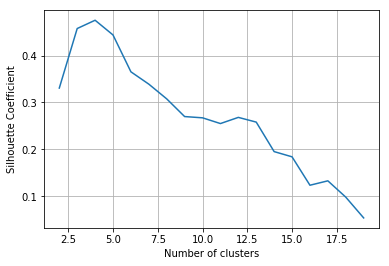In :
# K-means with 4 clusters on scaled data
km = KMeans(n_clusters=4, random_state=1)
km.fit(X_scaled)
beer['cluster'] = km.labels_
beer.sort_values('cluster')

Out:
name calories sodium alcohol cost cluster
0 Budweiser 144 15 4.7 0.43 0
1 Schlitz 151 19 4.9 0.43 0
17 Heilemans_Old_Style 144 24 4.9 0.43 0
16 Hamms 139 19 4.4 0.43 0
5 Old_Milwaukee 145 23 4.6 0.28 0
6 Augsberger 175 24 5.5 0.40 0
7 Srohs_Bohemian_Style 149 27 4.7 0.42 0
10 Coors 140 18 4.6 0.44 0
15 Pabst_Extra_Light 68 15 2.3 0.38 1
12 Michelob_Light 135 11 4.2 0.50 1
11 Coors_Light 102 15 4.1 0.46 1
9 Budweiser_Light 113 8 3.7 0.40 1
8 Miller_Lite 99 10 4.3 0.43 1
18 Olympia_Goled_Light 72 6 2.9 0.46 1
19 Schlitz_Light 97 7 4.2 0.47 1
13 Becks 150 19 4.7 0.76 2
14 Kirin 149 6 5.0 0.79 2
4 Heineken 152 11 5.0 0.77 2
3 Kronenbourg 170 7 5.2 0.73 2
2 Lowenbrau 157 15 0.9 0.48 3

## Part 3: DBSCAN clustering¶

In :
# DBSCAN with eps=1 and min_samples=3
from sklearn.cluster import DBSCAN
db = DBSCAN(eps=1, min_samples=3)
db.fit(X_scaled)

Out:
DBSCAN(algorithm='auto', eps=1, leaf_size=30, metric='euclidean',
metric_params=None, min_samples=3, n_jobs=1, p=None)
In :
# review the cluster labels
db.labels_

Out:
array([ 0,  0, -1,  1,  1, -1, -1,  0,  2,  2,  0,  2,  0, -1,  1, -1,  0,
0, -1,  2])
In :
# save the cluster labels and sort by cluster
beer['cluster'] = db.labels_
beer.sort_values('cluster')

Out:
name calories sodium alcohol cost cluster
2 Lowenbrau 157 15 0.9 0.48 -1
5 Old_Milwaukee 145 23 4.6 0.28 -1
6 Augsberger 175 24 5.5 0.40 -1
18 Olympia_Goled_Light 72 6 2.9 0.46 -1
13 Becks 150 19 4.7 0.76 -1
15 Pabst_Extra_Light 68 15 2.3 0.38 -1
0 Budweiser 144 15 4.7 0.43 0
1 Schlitz 151 19 4.9 0.43 0
7 Srohs_Bohemian_Style 149 27 4.7 0.42 0
17 Heilemans_Old_Style 144 24 4.9 0.43 0
10 Coors 140 18 4.6 0.44 0
16 Hamms 139 19 4.4 0.43 0
12 Michelob_Light 135 11 4.2 0.50 0
3 Kronenbourg 170 7 5.2 0.73 1
4 Heineken 152 11 5.0 0.77 1
14 Kirin 149 6 5.0 0.79 1
9 Budweiser_Light 113 8 3.7 0.40 2
8 Miller_Lite 99 10 4.3 0.43 2
11 Coors_Light 102 15 4.1 0.46 2
19 Schlitz_Light 97 7 4.2 0.47 2
In :
# review the cluster centers
beer.groupby('cluster').mean()

Out:
calories sodium alcohol cost
cluster
-1 127.833333 17.0 3.483333 0.460000
0 143.142857 19.0 4.628571 0.440000
1 157.000000 8.0 5.066667 0.763333
2 102.750000 10.0 4.075000 0.440000
In :
# scatter plot matrix of DBSCAN cluster assignments (0=red, 1=green, 2=blue, -1=yellow)
pd.scatter_matrix(X, c=colors[beer.cluster], figsize=(10,10), s=100)

/Users/georgioskarakostas/anaconda3/lib/python3.6/site-packages/ipykernel_launcher.py:2: FutureWarning: pandas.scatter_matrix is deprecated, use pandas.plotting.scatter_matrix instead


Out:
array([[<matplotlib.axes._subplots.AxesSubplot object at 0x1a18b05518>,
<matplotlib.axes._subplots.AxesSubplot object at 0x1a18b3ae48>,
<matplotlib.axes._subplots.AxesSubplot object at 0x1a18bd1518>,
<matplotlib.axes._subplots.AxesSubplot object at 0x1a18bf9ac8>],
[<matplotlib.axes._subplots.AxesSubplot object at 0x1a18c2a160>,
<matplotlib.axes._subplots.AxesSubplot object at 0x1a18c2a198>,
<matplotlib.axes._subplots.AxesSubplot object at 0x1a18c7ae80>,
<matplotlib.axes._subplots.AxesSubplot object at 0x1a18cac550>],
[<matplotlib.axes._subplots.AxesSubplot object at 0x1a18cd3be0>,
<matplotlib.axes._subplots.AxesSubplot object at 0x1a18d072b0>,
<matplotlib.axes._subplots.AxesSubplot object at 0x1a18d2d940>,
<matplotlib.axes._subplots.AxesSubplot object at 0x1a18d57fd0>],
[<matplotlib.axes._subplots.AxesSubplot object at 0x1a18d866a0>,
<matplotlib.axes._subplots.AxesSubplot object at 0x1a18db1d30>,
<matplotlib.axes._subplots.AxesSubplot object at 0x1a18de1400>,
<matplotlib.axes._subplots.AxesSubplot object at 0x1a18e09a90>]],
dtype=object)